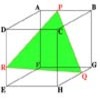#### You may also like### Cut Cube

Find the shape and symmetries of the two pieces of this cut cube.### Maximum Scattering

Your data is a set of positive numbers. What is the maximum value that the standard deviation can take?

# Walls

##### Age 16 to 18 Challenge Level:

Let $P_1$ be a plane through the points $A(2,1,0), B(1,1,1)$ and $C(1,7,3)$ and $P_2$ be a plane through the points $A$, $B$ and $V(x,y,z)$. Find all the points $V(x,y,z)$ such that the two planes are perpendicular.